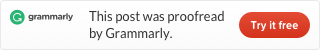Categories

# LeetCode 232. Implement Queue using Stacks (Java)

Implement the following operations of a queue using stacks.

• push(x) — Push element x to the back of queue.
• pop() — Removes the element from in front of queue.
• peek() — Get the front element.
• empty() — Return whether the queue is empty.

Example:

```MyQueue queue = new MyQueue();

queue.push(1);
queue.push(2);
queue.peek();  // returns 1
queue.pop();   // returns 1
queue.empty(); // returns false```

Notes:

• You must use only standard operations of a stack — which means only `push to top``peek/pop from top``size`, and `is empty` operations are valid.
• Depending on your language, stack may not be supported natively. You may simulate a stack by using a list or deque (double-ended queue), as long as you use only standard operations of a stack.
• You may assume that all operations are valid (for example, no pop or peek operations will be called on an empty queue).

This problem is the perfect opposite of problem 225, and the solution is almost identical. Other parts just use methods of the stack. The push method, we create a temporary stack to add new value, then add all the elements of the old stack, then use the new stack as internal storage.

Other problems related to stack and queue, see also Stack and Queue Data Structure (Java code and Leetcode problems)This site uses Akismet to reduce spam. Learn how your comment data is processed.